ESGS Logical Fallacies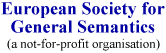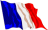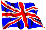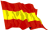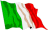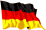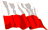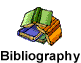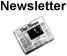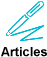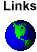Gambler's Fallacy

 Definition

The gambler's fallacy is the mistaken notion that the odds for something with a fixed probability increase or decrease depending upon recent occurrences.

 Explanation

This is a fallacy because mathematically wrong. With two independents events, such as two dice throwings, lottery numbers, etc., the result of the first event does not change the probability of the next.

 Examples

Last time, I made a 6 with that dice, so this time I'll try another number. The probability of ahving a six is as high as that of other numbers.

 Counter-examples
When events are not independent, the probabilities can change.